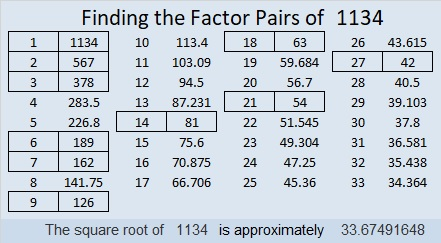# 1134 Some Likely Factor Trees

I could easily make nine factor trees for 1134 using different factor pairs of 1134. However, most people aren’t likely to begin a factor tree by first dividing 1134 by 14, 18, 21, or 27. Most people will want to start with a 1-digit divisor of 1134.

Most people will also use only one color of ink or pencil lead to make a factor tree.

Here are three of 1134’s factor trees that are much more likely to be made by the average person.How easy is it to find all the prime factors in those one-color trees? You will probably think it is easier for some trees than others.

I think the tree in the middle is the easiest to read. All the prime factors are in the correct order and easy to distinguish from the composite factors in the tree. Although it is similar to using the cake method, I still like the cake method better.

Here are some facts about the number 1134:

• 1134 is a composite number.
• Prime factorization: 1134 = 2 × 3 × 3 × 3 × 3 × 7, which can be written 1134 = 2 × 3⁴ × 7
• The exponents in the prime factorization are 1, 4 and 1. Adding one to each and multiplying we get (1 + 1)(4 + 1)(1 + 1) = 2 × 5 × 2 = 20. Therefore 1134 has exactly 20 factors.
• Factors of 1134: 1, 2, 3, 6, 7, 9, 14, 18, 21, 27, 42, 54, 63, 81, 126, 162, 189, 378, 567, 1134
• Factor pairs: 1134 = 1 × 1134, 2 × 567, 3 × 378, 6 × 189, 7 × 162, 9 × 126, 14 × 81, 18 × 63, 21 × 54, or 27 × 42
• Taking the factor pair with the largest square number factor, we get √1134 = (√81)(√14) = 9√14 ≈ 33.674921134 is the sum of four consecutive prime numbers:
277 + 281 + 283 + 293 = 1134

This site uses Akismet to reduce spam. Learn how your comment data is processed.